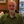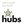0%
0%

step by step how to build your own Concurrently Multi Channels VFOs Memory Scanner Software using GNU-RADIO Companion under Win 10

Similar projects worth following
208 views

this is not jump scanning in different frequencies ranges , its SCANNING AT THE SAME Dongle Bandwidth Range , i build in this video the principle how to make scanning VFOs memory for TWO VFOs : VFO1+ VFO2 , and you can increase it as what you like .. i make my own 13 VFOs Scanning and works well too ! its very good for monitoring NFM analog frequencies if its all closer together and at your dongle bandwidth ... we learn how to make RF Gain slider , VFO1 , VFO2 , SQL , PPM Correction , Center frequency , volume ....i use in this long tutorial 32 BLOCKS .

Share

## Discussions

MWK wrote 07/04/2020 at 09:20 point

in this video step by step how to build your own Concurrently Multi Channels VFOs Memory Scanner Software using GNU-RADIO Companion under Win 10 , this is not jump scanning in different frequencies ranges , its SCANNING AT THE SAME Dongle Bandwidth Range , i build in this video the principle how to make scanning VFOs memory for TWO VFOs : VFO1+ VFO2 , and you can increase it as what you like .. i make my own 13 VFOs Scanning and works well too ! its very good for monitoring NFM analog frequencies if its all closer together and at your dongle bandwidth ... we learn how to make RF Gain slider , VFO1 , VFO2 , SQL , PPM Correction , Center frequency , volume ....i use in this long tutorial 32 BLOCKS .
1:00:53 screenshots with details of all 32 steps and with how to use grid position for sliders .
what Blocks i use : 1- wx gui main block 2- wx gui slider block : id=center_freq label= Center Frequency 3- variable block : id=down_rate value=250e3 4- variable block : id=samp_rate value=1e6 5- wx gui slider block : id=volume label=Vol. 6- wx gui slider block : id=rfgain 7- variable block : id=channel_width value=250e3 8- wx gui slider block : id=channel_freq1 label= VFO Freq 1 9- wx gui slider block : id=channel_freq2 label= VFO Freq 2
((( you can make many wx gui slider the same up as you like for VFO Freq 3 , VFO Freq 4 , ......)))
10- wx gui slider block : id=sql_lev label=SQL
11- wx gui slider block : id=ppm_correction label=PPM Corr.
12- osmocom source : sample rate=samp_rate ch0 frequency=center_freq ch0 freq corr=ppm_correction ch0 RF Gain=rfgain ch0 gain mode=manual
13- wx gui fft sink block : sample rate=samp_rate Baseband Freq=center_freq Average=on
14- multiply block : (for vfo 1 freq1) 15- signal source block : (for vfo 1 freq1) Sample Rate=samp_rate Frequency=center_freq - channel_freq1
16- multiply block : (for vfo 2 freq2)
17- signal source block : (for vfo 2 freq2) Sample Rate=samp_rate
Frequency=center_freq - channel_freq2
18- low pass filter block : (for vfo 1 freq1) FIR Type=Complex-Coplex(Decimating)
Decimation=int(samp_rate/channel_width) gain=1 Sample Rate=samp_rate Cutoff Freq=1e3 Transition Width=12.5e3 window=hamming
19- low pass filter block : (for vfo 2 freq2) FIR Type=Complex-Coplex(Decimating)
Decimation=int(samp_rate/channel_width) gain=1 Sample Rate=samp_rate Cutoff Freq=1e3 Transition Width=12.5e3 window=hamming
20- simple squelch block : (for vfo 1 freq1) Threshold= -50 Alpha= 1e-4
21- simple squelch block : (for vfo 2 freq2) Threshold= -50 Alpha= 1e-4
22- throttle block : (for vfo 1 freq1) Type=complex Sample Rate= samp_rate Vec=1
23- throttle block : (for vfo 2 freq2) Type=complex Sample Rate= samp_rate Vec=1
24- FM Demod block : (for vfo 1 freq1) Channel Rate= down_rate Audio Decimation=1
25- FM Demod block : (for vfo 2 freq2) Channel Rate= down_rate Audio Decimation=1
26- rational reasapler block : (for vfo 1 freq1) Type=Float-Float (Real Taps)
interpolation=24 decimation=250
27- rational reasapler block : (for vfo 2 freq2) Type=Float-Float (Real Taps)
interpolation=24 decimation=250
28- multiply const block : (for vfo 1 freq1) IO Type=float Constant=volume/100 Vec=1
29- multiply const block : (for vfo 2 freq2) IO Type=float Constant=volume/100 Vec=1
30- add block : IO Type= float Num Inputs =2 Vec=1 (((( if you have like 6 VFOs you need to change the add block Num Inputs =6 and so on )))) ( we have two VFOs so the ADD Block Num Inputs = 2 )
31- power squelch block : Type=float Threshold= sql_lev Alpha=1 Ramp=1
32- audio sink block : Saple Rate= 24khz

(( TO ADD MANY VFOs for Scanning just repeat steps : 14 , 15 , 18 , 20 , 22 , 24 , 26 , 28 , 30 (add number of inputs as required ) and define new wx gui slider like step 8 and step 9 for VFO Freq 3 and more ..... thanks ................ MWK ................

Are you sure? yes | no

## Similar Projects

Project Owner Contributor

### Signal Processing techniques for BeginnersPhilip

Project Owner Contributor

### hubs = geodesic domes made simplebuild with hubs

# Does this project spark your interest?

Become a member to follow this project and never miss any updates